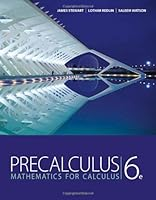# Precalculus: Mathematics for Calculus, 6th Edition## Book Description

This best selling author team explains concepts simply and clearly, without glossing over difficult points. Problem solving and are introduced early and reinforced throughout, providing students with a solid foundation in the principles of mathematical thinking. Comprehensive and evenly paced, the book provides complete coverage of the function concept, and integrates a significant amount of graphing calculator material to help students develop insight into mathematical ideas. The authors' attention to detail and clarity, the same as found in James Stewart's market-leading Calculus text, is what makes this text the market leader.

Chapter 1:
Chapter 2:
Chapter 3: Polynomial And Functions
Chapter 4: Exponential And Logarithmic Functions
Chapter 5: Trigonometric Functions: Unit Circle Approach
Chapter 6: Trigonometric Functions: Right Triangle Approach
Chapter 7: Analytic Trigonometry
Chapter 8: Polar Coordinates And Parametric Equations
Chapter 9: Vectors In Two And Three Dimensions
Chapter 10: Of Equations And Inequalities
Chapter 11: Conic Sections
Chapter 12: Sequences And Series
Chapter 13: Limits: A Preview Of Calculus
Appendix: Calculations And Significant Figures